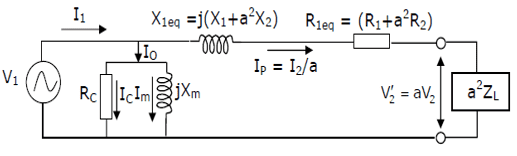# Calculating Transformer Input Voltage

## Homework Statement

Calculate the primary current, and hence the voltage at the transformer input winding, V1.

Transformation ratio, a = N1/N2 = 0.1
R1 = 0.12 Ω; R2 = 12 Ω
X1 = 0.4 Ω ; X2 = 40 Ω
RC = 560 Ω
V2 = 2300 V
RL = 1 kΩ
'Xm = 800 Ω'

## Homework Equations

R1eq = R1 + a2R2
X1eq = j(X1 + a2X2)
V2' = aV2

Current divider equation.

## The Attempt at a Solution

[/B]
Approximate equivalent circuit:R1eq = 0.12 + (0.1)2(12)
R1eq = 0.24 Ω

X1eq = j(0.4 + (0.1)2(40))
X1eq =j0.8 Ω

V2' = 0.1(2300)
V2' = 230 V

a2ZL = (0.1)2(1000)
a2ZL = 10 Ω

Ip = V2'/a2ZL
Ip = 23 A

From here is where I believed that there is likely a more efficient way to solve the problem -- particularly because the value of Xm was not actually given in the paper, but told to us during the tutorial, more-or-less made up on the spot.

Here is the outline of the given solution:

Ip= I1*Z2/(Z1+Z2)

⇒I1 = Ip*(Z1+Z2)/Z2

⇒I1 = 23*(Rc // Xm + X1eq + R1eq + a2ZL)/ (Rc // Xm)

I0 = I1 - Ip

∴ V1 = I0(Rc // Xm)

I'd be thankful for any input.

Hesch
Gold Member
From here is where I believed that there is likely a more efficient way to solve the problem

It seems that in the given solution another equivalent is used (T-equivalent) than the one in #1. It's hard to explain what happens in that other equivalent because I cannot see what is meant by Z1 and Z2.

Don't you like to calculate with complex numbers? I don't know if you find complex calculations "more efficient", but they will be more structured. Example:

Z0 = (Rc || jXm) = (560 || j800) = (375.8 + j263). That's it.

In the same way you calculate other impedances, that can be used in Kirchhoffs laws, etc.

It becomes simple as in the given solution: Ip= I1*Z2/(Z1+Z2). As for the numerical calculation I assume a calculator can do the job for you.

It seems that in the given solution another equivalent is used (T-equivalent) than the one in #1. It's hard to explain what happens in that other equivalent because I cannot see what is meant by Z1 and Z2.

Don't you like to calculate with complex numbers? I don't know if you find complex calculations "more efficient", but they will be more structured. Example:

Z0 = (Rc || jXm) = (560 || j800) = (375.8 + j263). That's it.

In the same way you calculate other impedances, that can be used in Kirchhoffs laws, etc.

It becomes simple as in the given solution: Ip= I1*Z2/(Z1+Z2). As for the numerical calculation I assume a calculator can do the job for you.

Hi,